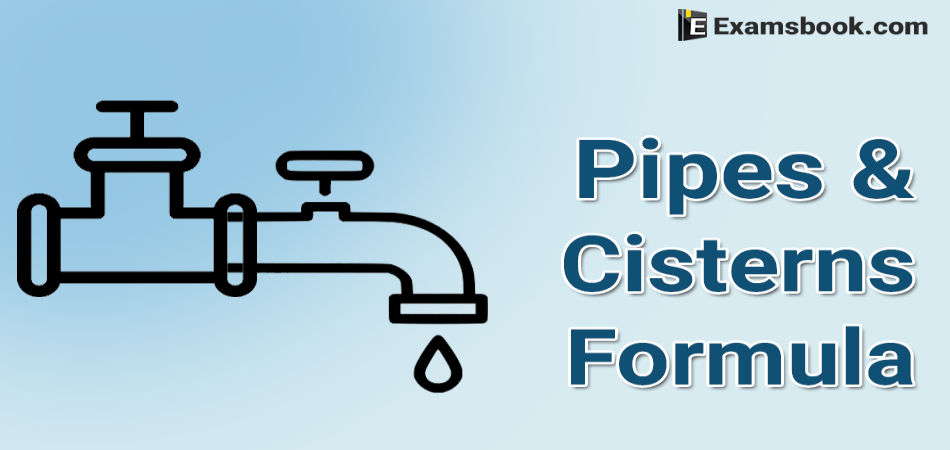• Save

# Pipes and Cisterns Formula for Competitive Exams

2 months ago 615 Views
NEWPipes and Cistern questions are important for SSC and Bank exams. But some of the students are facing problems while solving these questions in the examination. So, keeping the same problem in mind, I am sharing you pipes and cistern formulas for competitive exams.

With these formulas, you can know how to use formulas in pipe and cistern questions while solving. Let's start solving Pipe and Cistern questions yourself using these formulas for better results.

You can also practice with Pipes and cisterns Problems in Hindi.

## Pipes and Cisterns Formulas for Practice:

Inlet : A pipe connected with a tank or a cisterns or a reservoir, that fills it, is known as an inlet.

Outlet: A pipe connected with a tank or a cisterns or a reservoir, emptying it, is known as an outlet.

2. (I) If pipe can fill a tank in x hours, then :

Part filled in 1 hour = 1/x

(II) If a pipe can empty a full tank in y hours, then :

Part emptied in 1 hour = 1/y

(III) If a pipe can fill a tank in x hours and another pipe can empty the full tank in y hours (where y>x), then on opening both the pipes, the net part filled in 1 hours = [(1/x)-(1/y)].

(IV) If a pipe can fill a tank in x hours and another pipe can empty the full tank in y hours (where x>y), then on opening both the pipes, the net part emptied in 1 hours = [(1/y) – (1/x).

Some example:

Ex. Two pipes can fill a tank in 10 hours and 12 hours respectively while a third pipe empties the full tank in 20 hours. If all the three pipes operate simultaneously, in how much time will the tank be filled?

Solution:

Net part filled in 1 hours = [(1/10)+(1/12)-(1/20)]= 8/60 = 2/15.

The tank will be full in 15/2 hrs = 7 hrs 30 min.

Ex. A cisterns has two taps which fill it in 12 minutes and 15 minutes respectively. There is also a waste pipe in the cistern. When all the three are opened, the empty cistern is full in 20 minutes. How long will the waste pipe take to empty the full cistern?

Solution:

Work done by the waste pipe in 1 minute

= [(1/20)-{(1/12+1/15)= - 1/10. [ - ve sign means emptying]

Waste pipe will empty the full cistern in 10 minutes.

Ex. Two pipes A and B can fill a tank in 20 and 30 minutes respectively. If both the pipes are used together, then how long will it take to fill the tank?

Solution:

Part filled by A in 1 min = 1/20; Part filled by B in 1 min. = 1/30.

Part filled by (A+B) in 1 min. = [(1/20)+(1/30)]=1/12.

Both the pipes can fill the tank in 12 minutes.

Ex. A tap can fill a tank in 6 hours. After the tank is filled, three more similar taps are opened. What is the total time taken to fill the tank completely?

Solution

Time taken by one tap to fill half the tank = 3 hrs.

Part filled by the four taps in 1 hours = [4×(1/6)] =2/3

Remaining Part = [1-(1/2)] = 1/2.

⸫ 2/3: 1/2 :: 1: x

Or  x=[(1/2)×1×(3/2)] = 3/4 hrs i.e, 45 mins.

So, total time taken = 3 hrs 45 min.

Ask me in the comment section without any hesitation if you have any problem or doubt regarding pipes and cistern formulas.

## About author

### Vikram Singh

Providing knowledgable questions of Reasoning and Aptitude for the competitive exams.

## Report Error : Pipes and Cisterns Formula for Competitive Exams

Please Enter Message
Error Reported Successfully